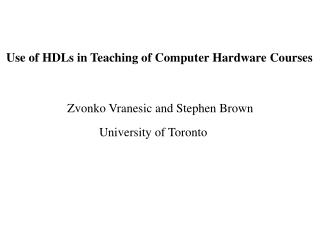# Use of HDLs in Teaching of Computer Hardware Courses - PowerPoint PPT PresentationDownload PresentationUse of HDLs in Teaching of Computer Hardware Courses

Use of HDLs in Teaching of Computer Hardware Courses
Download Presentation## Use of HDLs in Teaching of Computer Hardware Courses

- - - - - - - - - - - - - - - - - - - - - - - - - - - E N D - - - - - - - - - - - - - - - - - - - - - - - - - - -
##### Presentation Transcript

1. Use of HDLs in Teaching of Computer Hardware Courses Zvonko Vranesic and Stephen Brown University of Toronto

2. Message of this talk • Introduce Verilog or VHDL early • Integrate the discussion of logic circuits and HDL representations • Course becomes more interesting and useful

3. Typical course sequence . . . Logic Design Computer Organization . . .

4. Key points • HDL is not a programming language • Start with a structural approach • Make sure that students see wires and flip-flops • Progress to behavioral approach • Explain the impact of target technology

5. The next few slides show how a number of Verilog concepts can be introduced using an example of a ripple-carry adder. This approach is used in the book: S. Brown and Z. Vranesic: “Fundamentals of Digital Logic with Verilog Design” McGraw-Hill, 2003

6. x y x y x y 1 1 0 0 n – 1 n – 1 c 1 c c c c FA FA FA n - 1 n 0 2 s s s n – 1 1 0 MSB position LSB position An n-bit ripple-carry adder.

7. module fulladd (Cin, x, y, s, Cout); input Cin, x, y; output s, Cout; assign s = x ^ y ^ Cin; assign Cout = (x & y) | (x & Cin) | (y & Cin); endmodule Verilog code for the full-adder.

8. module adder4 (carryin, X, Y, S, carryout); input carryin; input [3:0] X, Y; output [3:0] S; output carryout; wire [3:1] C; fulladd stage0 (carryin, X, Y, S, C); fulladd stage1 (C, X, Y, S, C); fulladd stage2 (C, X, Y, S, C); fulladd stage3 (C, X, Y, S, carryout); endmodule A four-bit adder.

9. module addern (carryin, X, Y, S, carryout); parameter n=32; input carryin; input [n-1:0] X, Y; output [n-1:0] S; output carryout; reg [n-1:0] S; reg carryout; reg [n:0] C; integer k; always @(X or Y or carryin) begin C = carryin; for (k = 0; k < n; k = k+1) begin S[k] = X[k] ^ Y[k] ^ C[k]; C[k+1] = (X[k] & Y[k]) | (X[k] & C[k]) | (Y[k] & C[k]); end carryout = C[n]; end endmodule A generic specification of a ripple-carry adder.

10. module addern (carryin, X, Y, S); parameter n = 32; input carryin; input [n-1:0] X, Y; output [n-1:0] S; reg [n-1:0] S; always @(X or Y or carryin) S = X + Y + carryin; endmodule Specification of an n-bit adder using arithmetic assignment.

11. module addern (carryin, X, Y, S, carryout, overflow); parameter n = 32; input carryin; input [n-1:0] X, Y; output [n-1:0] S; output carryout, overflow; reg [n-1:0] S; reg carryout, overflow; always @(X or Y or carryin) begin S = X + Y + carryin; carryout = (X[n-1] & Y[n-1]) | (X[n-1] & ~S[n-1]) | (Y[n-1] & ~S[n-1]); overflow = carryout ^ X[n-1] ^ Y[n-1] ^ S[n-1]; end endmodule An n-bit adder with carry-out and overflow signals.

12. module addern (carryin, X, Y, S, carryout, overflow); parameter n = 32; input carryin; input [n-1:0] X, Y; output [n-1:0] S; output carryout, overflow; reg [n-1:0] S; reg carryout, overflow; reg [n:0] Sum; always @(X or Y or carryin) begin Sum = {1'b0,X} + {1'b0,Y} + carryin; S = Sum[n-1:0]; carryout = Sum[n]; overflow = carryout ^ X[n-1] ^ Y[n-1] ^ S[n-1]; end endmodule A different specification of an n-bit adder with carry-out and overflow signals.

13. module addern (carryin, X, Y, S, carryout, overflow); parameter n = 32; input carryin; input [n-1:0] X, Y; output [n-1:0] S; output carryout, overflow; reg [n-1:0] S; reg carryout, overflow; always @(X or Y or carryin) begin {carryout, S} = X + Y + carryin; overflow = carryout ^ X[n-1] ^ Y[n-1] ^ S[n-1]; end endmodule Simplified complete specification of an n-bit adder.

14. module fulladd (Cin, x, y, s, Cout); input Cin, x, y; output s, Cout; reg s, Cout; always @(x or y or Cin) {Cout, s} = x + y + Cin; endmodule Behavioral specification of a full-adder.

15. Final comment Students at University of Toronto have responded very positively to this approach.